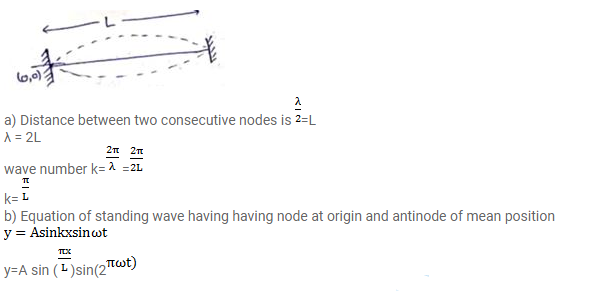# A string of length L fixed at both ends vibrates in its fundamentalQuestion:

A string of length $L$ fixed at both ends vibrates in its fundamental mode at a frequency $v$ and a maximum amplitude A.

a. Find the wavelength and the wave number $k$.

b. Take the origin at one end of the string and the $X$-axis along the string. Take the $Y$-axis along the direction of the displacement. Take $\mathrm{t}=0$ at the instant when the middle point of the string passes through its mean position and is going towards the positive $y$-direction. Write the equation describing the standing wave.

Solution: